#### Chapter 1 Number System R.D. Sharma Solutions for Class 9th MCQ's

Mark the correct alternative in each of the following:

1. Which one of the following is a correct statement?
(a) Decimal expansion of a rational number is terminating
(b) Decimal expansion of a rational number is non-terminating
(c) Decimal expansion of an irrational number is terminating
(d) Decimal expansion of an irrational number is non-terminating and non-repeating.

Solution

The decimal expansion of an irrational number is non-terminating and non- repeating. Thus, we can say that a number, whose decimal expansion is non-terminating and non- repeating, called irrational number. And the decimal expansion of rational number is either terminating or repeating. Thus, we can say that a number, whose decimal expansion is either terminating or repeating, is called a rational number. Hence the correct option is d.

2. Which one of the following statements is true? (a) The sum of two irrational numbers is always an irrational number
(b) The sum of two irrational numbers is always a rational number
(c) The sum of two irrational numbers may be a rational number or an irrational number
(d) The sum of two irrational numbers is always an integer

Solution

Since, 2 and 2 - 1 are two irrational number and -2 + (2+1) = 1
Therefore, sum of two irrational number may be rational
Now, let 3 and 2 - 3 be two irrational numbers and 3 + (2 - 3) = 2
Therefore, sum of two irrational number may be irrational
Hence the correct option is c.

3. Which of the following is a correct statement?
(a) Sum of two irrational numbers is always irrational
(b) Sum of a rational and irrational number is always an irrational number
(c) Square of an irrational number is always a rational number
(d) Sum of two rational numbers can never be an integer

Solution

The sum of irrational number and rational number is always irrational number .
Let a be a rational number and b be an irrational number.

Then,
Let a be a rational number and b be an irrational number.
Then,
(a+b)2 = a2 + b2 + 2ab
= (a2 + b2) + 2ab
As 2ab is irrational number therefore (a+b)2 is irrational .
Hence (a+b) is irrational .

4 . Which of the following statements is true?
(a) Product of two irrational numbers is always irrational.
(b) Product of a rational and an irrational number is always irrational.
(c) Sum of two irrational numbers can never be irrational.
(d) Sum of an integer and a rational number can never be an integer.

Since, we know that the product of rational and irrational number is always an irrational.
For example : Let 1/2, 3 are rational and irrational number respectively and their product is √3/2.
Hence the correct option is b .

5. Which of the following is irrational ?Solution6. Which of the following is irrational?
(i) 0.14
(ii) 0.1416
(iii) 0.1416
(iv) 0.1014001400014

Solution

Given that,
0.14:

0.1416
0.1416
• 0.1014001400014.... is not terminating or non-repeating. So, it is an irrational number.
Hence, the correct option is d.

7. Which of the following is rational ?
(a) 3
(b) π
(c) 4/10
(d) 0/4

Solution

Given that,
3, π , 4/10 and 0/4
Here,  0/4 = 0, this is the form of p/q . so this is a rational number
Hence the correct option is d.

8. The number 0.318564318564318564 ........ is:
(a) a natural number
(b) an integer
(c) a rational number
(d) an irrational number

Solution

Since, the given number 0.318564318564318564.... = 0.318564 is repeating, so it is a rational number because rational number is always either terminating or repeating. Hence, the correct option is d.

9. If n is a natural number, then √n
(a) always a natural number
(b) always an irrational number
(c) always an irrational number
(d) sometimes a natural number and sometimes an irrational number

Solution

The term “natural number” refers either to a member of the set of positive integer. 1,2,3. And natural number starts from one of counting digit .Thus, if n is a natural number then sometimes n is a perfect square and sometimes it is not. Therefore, sometimes is a natural number and sometimes it is an irrational number  Hence the correct option is d .

10. Which of the following numbers can be represented as non-terminating, repeating decimals ?
(a) 39/24
(b) 3/16
(c) 3/11
(d) 137/25

Solution

Given that
39/24 = 1.625
3/16 = 0.1875
3/11 = 0.272727272
1.37/25 = 0.0548
Here 3/11 is repeating but non-terminating.
Hence the correct option is c.

11. Every point on a number line represents
(a) a unique real number
(b) a natural number
(c) a rational number
(d) an irrational number

Solution

In basic mathematics, number line is a picture of straight line on which every point is assumed to correspond to real number.
Hence the correct option is a.

12. An irrational number between 2 and 2.5 is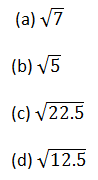Solution

Let
a=2
b=2.5
Here a and b are rational numbers. So we observe that in first decimal place a and b have distinct. According to question a < b.so an irrational number between 2 and 2.5 is 2.236067978 OR  √5
Hence the correct answer is b.

13. Which of the following is irrational ?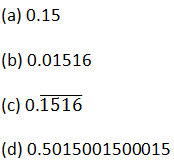Solution

Given,
Decimal number are:
0.15, 0.1516, and 0.5015000115....
Here, the number 0.5015000115.... is non terminating or non-repeating.
Hence, the correct option is d.

14. The number of consecutive zeros in 23 ×34 ×54 ×7 is
(a) 3
(b) 2
(c) 4
(d) 5 Solution

We are given the following expression and asked to find out the number of consecutive zeros
23×34×54×7
We basically, will focus on the powers of 2 and 5 because the multiplication of these two number gives one zero. So
23×34×54×7=23×54×34×7
=23×53×5×34×7
=(2×5)3×5×34×7
=103×5×34×7
=5×81×7×1000
=2835000
Therefore the consecutive zeros at the last is 3
So the option (a) is correct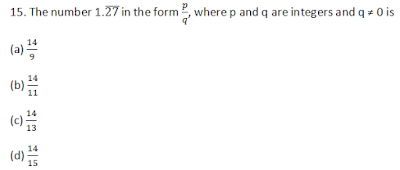SolutionSolution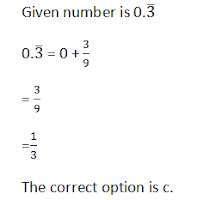17. The smallest rational number by which 1/3 should be multiplied so that its decimal expansion terminate after one place of decimal, is
(a) 1/10
(b) 3/10
(c)  3
(d) 30

SolutionSolution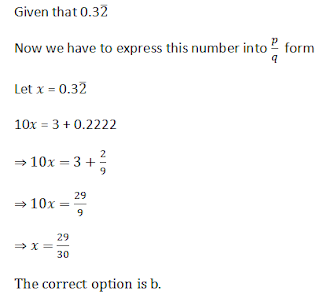Solution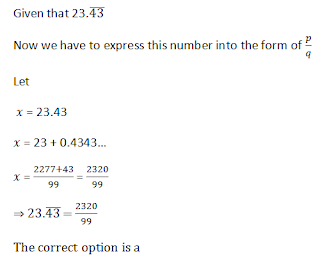Solution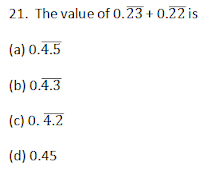Solution